# Applying OCR Technology for Receipt Recognition

by Ivan Ozhiganov

7 Apr 2016

The problem of optical character recognition (OCR) in various conditions remains as relevant today as it was in past years. Automated recognition of documents, credit cards, recognizing and translating signs on billboards — all of this could save time for collecting and processing data. With the development of convolutional neural networks (CNN) and methods of machine learning, the quality of text recognition is continually growing.

Having implemented a project for receipt recognition, we once again saw how effective convolutional neural networks are. For our research, we chose different receipts from a range of Russian stores with the text using both Cyrillic and Latin letters. The developed system can be easily adapted for recognizing receipts from other countries and text in other languages. Let’s consider the project in detail in order to demonstrate the operating principle of the solution.

The goal of our project is to develop an app using the client-server architecture for receipt recognition and extracting meaning from receipts.

## Project Overview

This task is broken into several stages:

• Preprocessing
— Finding a receipt on the image
— Binarization
• Finding the text
• Recognition
• Extracting meaning from receipts

## Implementation

### 1. Preprocessing

The preprocessing stage consists of the following preliminary work with the image: finding a receipt in the image, rotating the image so that the receipt strings are located horizontally, and then making a binarization of the receipt.

1.1. Rotating image to recognize a receipt
In order to solve the problem of finding a receipt in the image, we used the following methods:

• Adaptive binarization with a high threshold
• Convolutional Neural Network
• Finding a receipt via adaptive binarization with a high thresholdThe problem here was reduced to finding an area in the image that contains a complete receipt and minimum background.

In order to make the finding process simpler, we first rotate the image so that each individual row is as close to horizontal as possible (Image 2). The turning algorithm is required to maximize the variance of the brightness sum over the strings. The maximum is reached when the strings are located horizontally.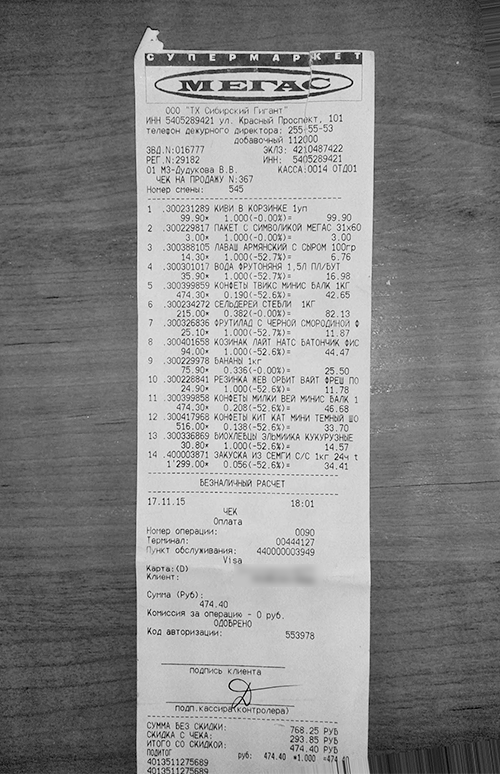We used the adaptive_threshold function from the library scikit-image to find the receipt. This function does adaptive binarization with a high threshold and leaves white pixels in areas with a high gradient, whereas areas that are more homogeneous become black. Using this function, only a few white pixels remain with a homogeneous background and we look for them in the described rectangle. As a result, the derived rectangle includes the receipt area and a minimum of unnecessary background.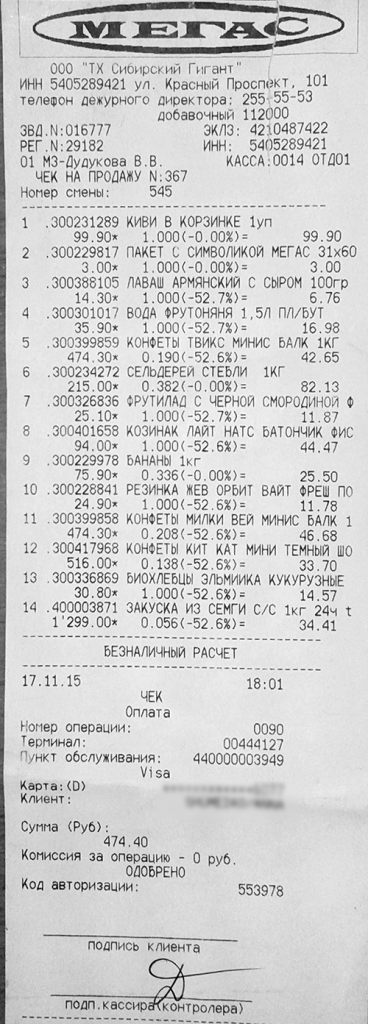### Finding a receipt using a convolutional neural network

We decided to find keypoints of the receipt using a convolutional neural network as we did it before for the object detection project. We chose the receipt angles as keypoints. This method performed well, although it was not as efficient as adaptive binarization with a high threshold.

The convolutional neural network showed a non-ideal result because it was trained for predicting the angle coordinates only relative to the found text. In addition to this the angles of the receipts meant that the text location varied from one receipt to another, and that’s why the precision of the CNN model is not very high.

Here are the results that the convolutional neural network demonstrated: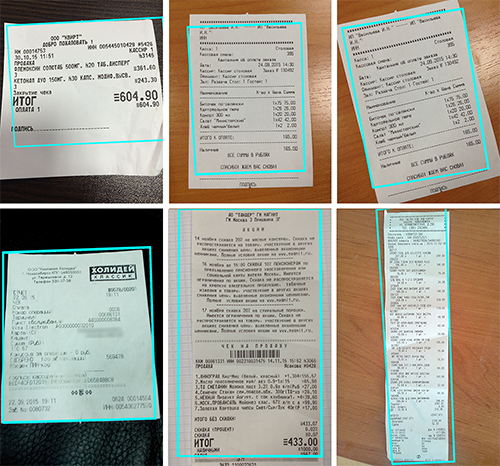### Finding a receipt using a Haar cascade classifier

As an alternative to the described models, we decided to try a Haar cascade classifier. After a week of training the Haar cascade classifier and changing parameters of receipt recognition, we didn’t get a satisfactory result. Even the CNN performed with higher quality.

Here are the examples of Haar cascade classifier work: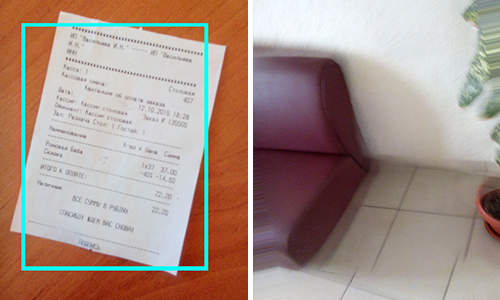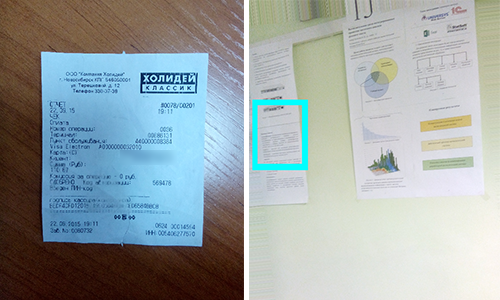1.2. Binarization

In the end we used the same adaptive_threshold method for binarization. The window is quite big so that it contains the text as well as the background.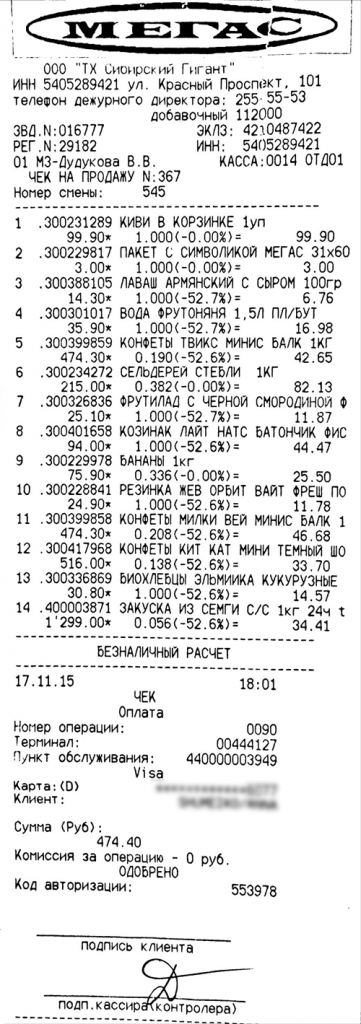### 2. Finding the text

2.1. Finding the text using the method of connected components

The first stage of finding the text consists of finding the connected components. We did this using the findContours function from OpenCV. The majority of connected components are real characters, but some of them are just noise fragments that are left after binarization. We eliminated them using filters across the maximal/minimal axis.

Then we applied the algorithm of combining connected components to the compound characters such as “:”, “Й”, “=”. After this the characters are combined to form words by searching their closest neighbors. The principle of a closest neighbors search is the following: it’s necessary to find the closest neighbors for every character, and then you can choose the most appropriate candidate for combination from the right and the left side. The algorithm continues until there are no more characters that do not belong to words.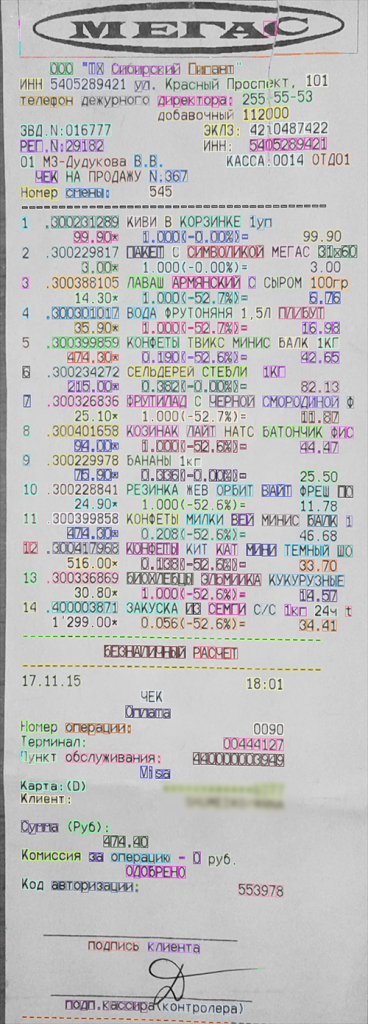Then lines are formed from the words. Here we use the theory that words are located at the same height (on the y-axis) in one line.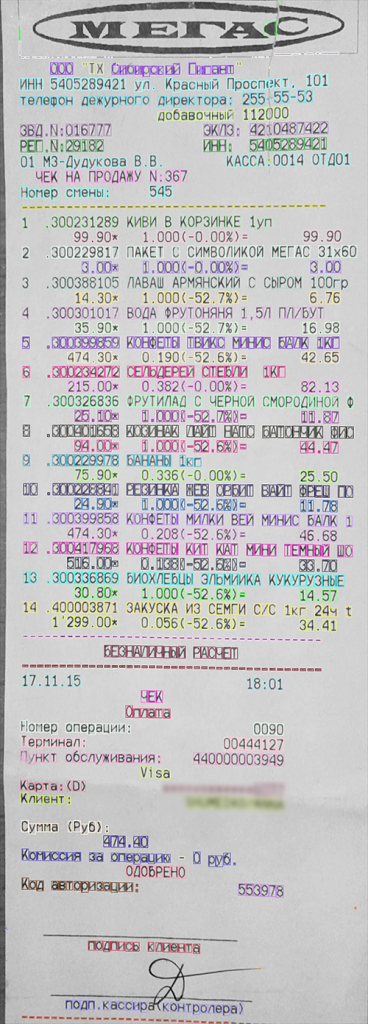The disadvantage is that this algorithm cannot properly recognize words with connected or broken letters.

2.2. Finding the text with a grid

We found that almost all the receipts have monospaced text. This means that we can draw a grid on the receipt in a way that all the grid lines go between the characters: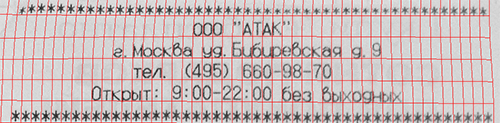An automatic search algorithm through a receipt grid simplifies further receipt recognition. A neural network can be applied to every cell of the grid and every character can be recognized. There are no complications with connected and broken characters. Finally, the number of spaces that goes side by side in the string is defined precisely.

We tried the following algorithm to find the described grid. First, you need to find connected components on the binary image:Then you should take the lower-left angles of the green rectangles and get the set of points that are given by coordinates. In order to determine distortions, we developed the 2d periodic function: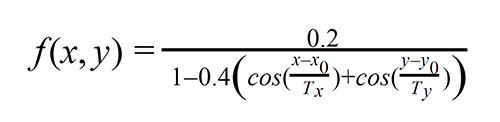The graph of the formula looks like this: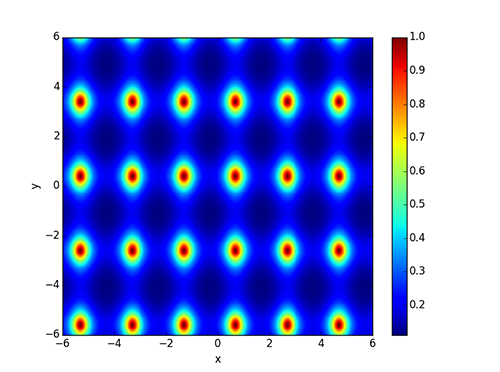The main idea behind the receipt grid is the finding of such nonlinear geometric distortions of the coordinates where the points are at the graph peaks. In other words, the problem is reformulated as a maximization problem of the function values sum. Taking this into account, it is necessary to find an optimal distortion. Geometric distortion was parametrized via the RectBivariateSpline function from the Scipy module in Python. Optimization was implemented using the minimize function from the Spicy module.

Here is an example of the correctly found grid: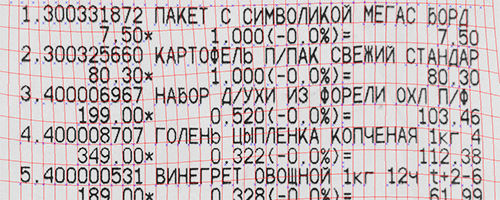Finally, we decided to avoid using this method because it has a range of significant drawbacks, is unstable, and is slow.

### 3. Optical Character Recognition (OCR)

3.1. Recognizing text found by the method of connected components

OCR is implemented by a convolutional neural network that was trained to find fonts taken from receipts. At the output, we have probabilities for every character and take several initial options that give us a probability close to 1 (99%) of the total sum. Then we consider all the possible options of compiling the words from the received characters and check them using a dictionary. It helps to improve the accuracy of recognition and to eliminate mistakes from similar characters (for example, "З" and "Э", Cyrillic alphabet).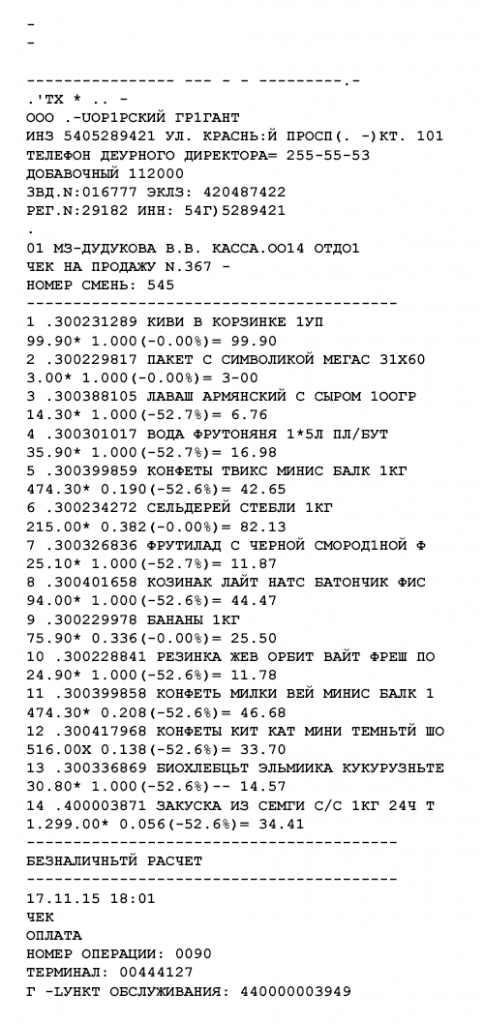Unfortunately, this method performs stably only when characters are not broken or connected between each other.

3.2. Recognition of complete words

It is required to recognize complete words in complicated cases when characters are broken or are connected between each other. We solved this problem using two methods:

• LSTM network
• Uniform segmentation

LSTM network

We decided to use an LSTM neural network to recognize complete words in complex cases in accordance with the articles devoted to the reading text in deep convolutional sequences and using LSTM networks for language-independent OCR. For this purpose, we used the library OCRopus.

We used monospaced fonts and prepared an artificial sample for training.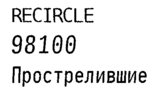After training the network we tested it with the validation set. Results of testing showed us that the network was trained well. Then we tested it using real receipts. Here are the results:The trained neural network performed well on simple examples that we successfully recognized using other methods previously. As for complex cases, the network didn’t work.

We decided to add various distortions to the training sample to approximate it to the words received in receipts.In order to avoid overfitting the network, we stopped the training process periodically, prepared a new dataset and continued training the network with the new dataset. Finally, we got the following results: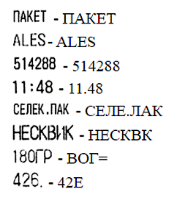The received neural network recognized complex words better but recognized simple words worse. As it was unstable, this model didn’t satisfy us.

We think that if you have a single font and minor distortions, this neural network could work much better.

Uniform Segmentation

The font on the receipts is monospaced. For this reason, we decided to split the words into characters uniformly. We need to know the character width inside the word. Thus, the mode of the character width was estimated for every receipt. If we have bimodal distribution of the character width, there are two modes chosen and the specific width is picked for every string.When we get an approximate character width in the string, we divide the length of the word by the character width and get the approximate number of characters. We then divide the length of the word by approximate number of characters plus or minus one: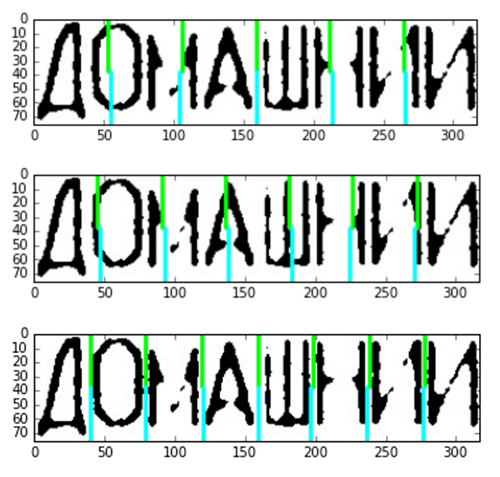Choosing the best option for division:The accuracy of such segmentation is quite high.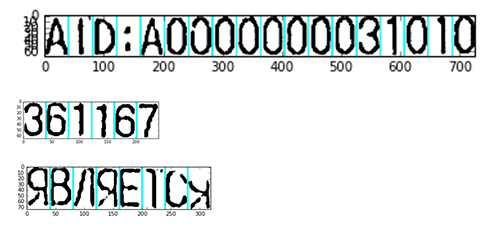However, sometimes we saw that the algorithm doesn’t work correctly:After segmentation, every fragment goes to the convolutional neural network where it is recognized.

### 4. Extracting meaning from receipts

The search of the purchases in a receipt is implemented by regular expressions. There is one common feature for all the receipts: the price of purchases is written in the XX.XX format, where X is a number. Therefore, it’s possible to extract the strings with purchases. The Individual Taxpayer Number can be found by 10 numbers and tested by the control sum. The Cardholder Name has the format NAME/SURNAME.## Conclusion

The problem of receipt recognition turned out to not be as simple as it seemed from first sight. While we looked for the solution, we faced many subproblems, that are fully or partially related to each other. Moreover, we understood that there is no silver bullet like we thought about LSTM. In practice, the majority of tools requires a lot of time for learning and don’t always become useful.

Our work on this project continues. We are concentrated on the improvement of quality for every stage of recognition and on the optimization of concrete algorithms. Currently, the system recognizes the receipts with very high precision if there are no connected or broken characters. When a receipt contains the connected or broken characters, the results are slightly worse than we expect.

• 0 SHARES